# Hperparameters | Regularization

## Regularization

Python代码为

for l in range(1, L):
L2_regularization_cost += (np.sum(np.square(Wl))
L2_regularization_cost = L2_regularization_cost * lambd/(2*m)


### Dropout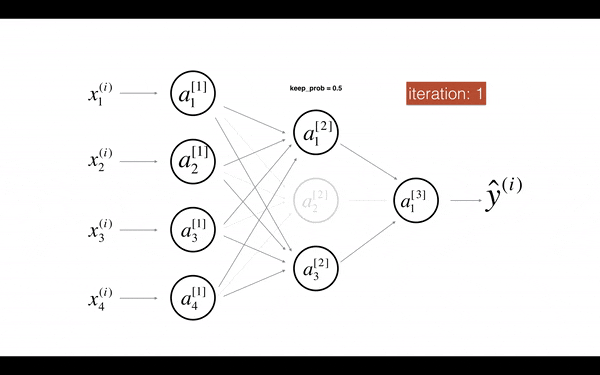Dropout使Activation units不依赖前面layer某些具体的unit，从而使模型更加泛化。在实际应用中，Dropout比较流行的实现是inverted dropout，其思路为

1. 产生一个bool矩阵, 以$l=3$为例
• d3 = (np.random.rand(a3.shape, a3.shape) < keep_prob).astype(int)
• 其中keep_prob表示保留某个hidden unit的概率。则d3是一个0和1的bool矩阵
2. 更新a3，根据keep_prob去掉某些units
• a3 = a3 * d3
3. Invert a3中的值。这么做相当于抵消掉去掉hidden units带来的影响
• a3 /= keep_prob

Numpy的伪代码如下

Z1 = np.dot(W1, X) + b1
A1 = relu(Z1)
D1 = np.random.rand(A1.shape, A1.shape)  # dropout matrix
D1 = (D1 < keep_prob).astype(int)  # dropout mask
A1 = A1*D1  # shut down some units in A1
A1 = A1/keep_prob  # scale the value of neurons that haven't been shut down


## Normalizing Training Sets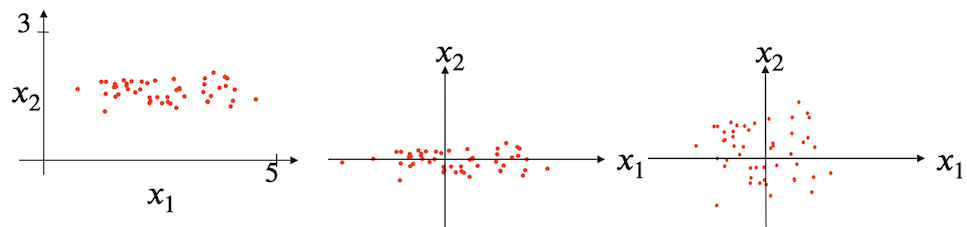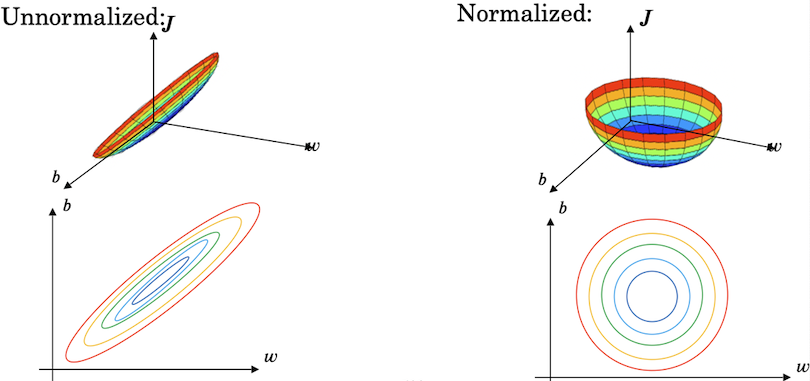### Weight Initialization for deep networksW[l] = np.random.rand(layers_dims[l]) * np.sqrt(2/(n**(l-1)))


Python的伪代码如下

def gradient_check(x, theta, epsilon = 1e-7):
thetaplus = theta + epsilon
thetaminus = theta - epsilon
J_plus = forward_propagation(x,thetaplus)
J_minus = forward_propagation(x,thetaminus)
gradapprox = (J_plus - J_minus) / (2*epsilon)

# Check if gradapprox is close enough to the output of backward_propagation()

difference = numerator / denominator

if difference < 1e-7:
else:
return difference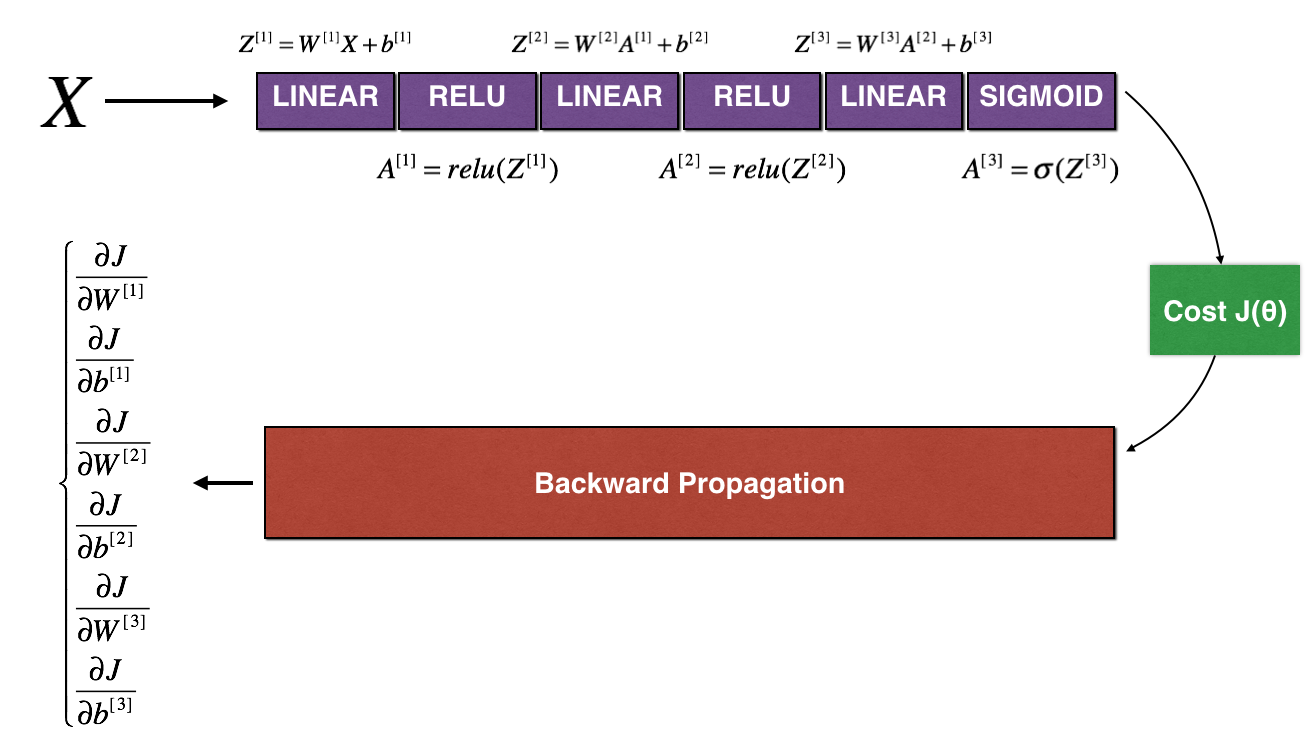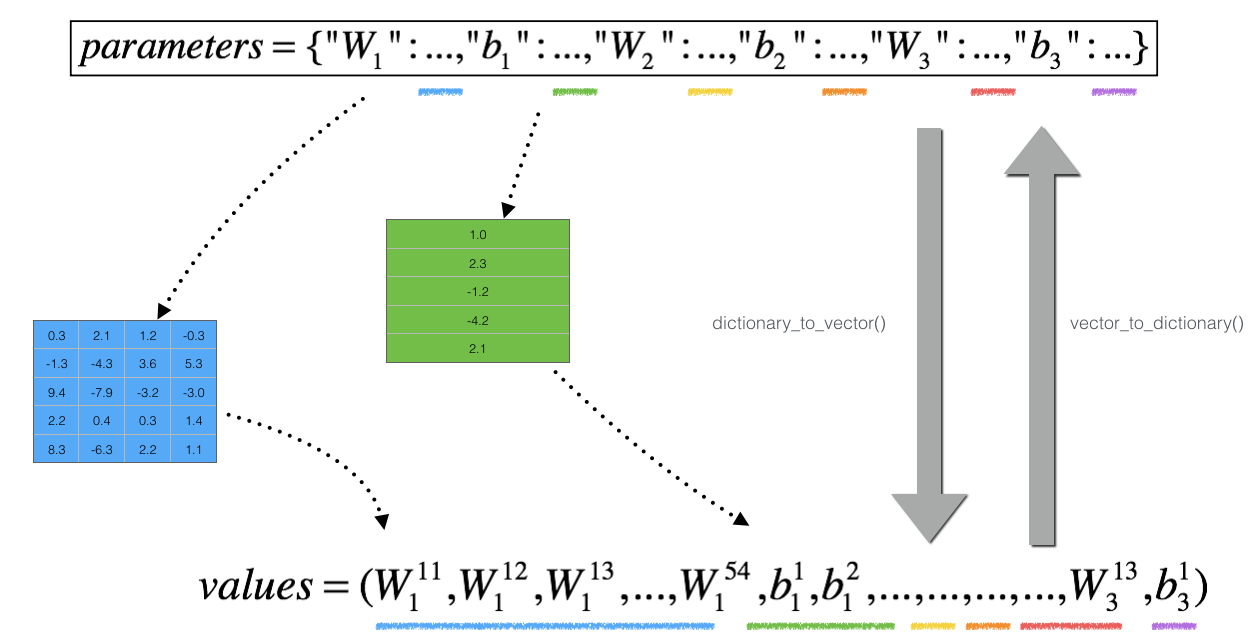1. 计算J_plus[i]
• 计算$\theta^{+}$ = np.copy(parameters_values)
• $\theta^{+} = \theta^{+} + \epsilon$
• $J^{+}_i$ = forward_propagation_n(x, y, vector_to_dictionary(theta_plus))
2. 重复上面步骤计算$\theta^{-}$和J_minus[i]
3. 计算导数值 $gradapprox[i] = \frac{J^{+}_i - J^{-}_i}{2 \varepsilon}[i] = \frac{J^{+}_i - J^{-}_i}{2 \varepsilon}$

• Note
• Gradient Checking is slow! Approximating the gradient with $\frac{\partial J}{\partial \theta} \approx \frac{J(\theta + \varepsilon) - J(\theta - \varepsilon)}{2 \varepsilon}$ is computationally costly. For this reason, we don’t run gradient checking at every iteration during training. Just a few times to check if the gradient is correct.
• Gradient Checking, at least as we’ve presented it, doesn’t work with dropout. You would usually run the gradient check algorithm without dropout to make sure your backprop is correct, then add dropout.